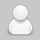2018
09-29

# 为什么Java中100==100为true而1000==1000为false？详细分析解释

这可能是每一个初学Java的人都会遇到的很有意思的问题。

执行下列代码：

```	Integer a = 100;
Integer b = 100;
Integer c = 1000;
Integer d = 1000;
System.out.println(a==b);
System.out.println(c==d);```

```true
false```

那么这是为什么呢？

这里我们就不得不提一下“==”的作用了(更多详情请点击链接了解)：我们都知道，“==”是用来比较两个变量是否相等，但是在具体应用中，基本类型和引用类型是要分开来讨论的，基本类型，如int、double、char等，使用“==”仅判断值是否相等，但是引用类型，如类和装箱值类型等，则比较的是两个变量引用的是否为同一个对象。

所以，看到这里，就应该可以理解为什么“c==d”返回的是false了，因为c和d是独立的两个对象。

等等！你是不是又有了新的疑问？为什么a==b返回的却是true呢？这不科学！很好，研究问题就是要将问题理解得足够透彻，要学会刨根问底，小伙子我看好你！

要弄清这个问题，我们可以打开Integer类的实现代码一探究竟（按住Ctrl鼠标左键点击Integer关键字即可打开实现代码）。下翻到代码约780行左右（jdk1.8）我们可以看到一个叫做“IntegerCache”的内部私有类，它缓存了从-128到127之间的所有的整数对象。

实际上，只有基本类型才支持直接赋值操作，而引用类型是需要使用“new”关键字来进行创建的，比如：

```	int a = 0;//基本类型
Integer b = new Integer(10);//引用类型```

但是，就如我们所使用的上述代码：

```	Integer a = 100;
Integer b = 100;
Integer c = 1000;
Integer d = 1000;```

为什么也能正常执行呢？

原来，是Java编译器在内部为我们进行了转换，自动调用了Integer类的“valueOf()”方法，当我们声明：

`	Integer a = 100;`

的时候，实际上执行的代码是：

`	Integer i = Integer.valueOf(100);`

不妨让我们来做个小实验：

```	Integer a = 100;
Integer b = 100;
Integer c = 1000;
Integer d = 1000;
Integer e = Integer.valueOf(100);
Integer f = Integer.valueOf(1000);
System.out.println(a==b);
System.out.println(c==d);
System.out.println(a==e);
System.out.println(c==f);```

输出结果为：

```true
false
true
false```

显然，和直接使用“=”定义得出的结果一致，可见我们的推理是正确的。

现在，让我们就来一起了解一下“valueOf()”函数的真面目：

```	public static Integer valueOf(int i) {
if (i >= IntegerCache.low && i <= IntegerCache.high)
return IntegerCache.cache[i + (-IntegerCache.low)];
return new Integer(i);
}```

可见，当赋值为“IntegerCache”中已经缓存的数字时，即-128~+127，Java将从高速缓存返回实例。

所以当我们执行：

```    Integer a = 100;
Integer b = 100;```

时，Java并不会重新创建对象，而是直接将高速缓存中的对象直接赋给a与b，即a与b指向同一个对象，所以“a==b”返回了“true”。你明白了吗？

有的朋友看到这里可能又要问了：为什么这里需要缓存？所有的数字统一处理多好？

一种比较合理的解释是，在此范围内的“小”整数比“大”整数的使用率要高，因此，使用相同的底层对象是有价值的，可以减少潜在的内存占用并提高执行效率。

那么，下面的代码的输出结果是什么呢？

```	Integer a = 100;
Integer g = new Integer(100);
Integer h = new Integer(100);
System.out.println(a==g);
System.out.println(g==h);```

哈哈，会不会有点头晕了呢？输出结果是：

```false
false```

直接new出来的当然是两个不同的对象啦，这里就不用再考虑高速缓存的问题了~

好了，本篇博客到此就要结束了，我们下篇再见。

行文参考：参考地址

```	public static void main(String[] args) {
doSomethingMagic();
int a = 2;
int b = a + a;
System.out.printf("%d + %d = %d", a, a, b); // 2 + 2 = 5
}

public static void doSomethingMagic() {
Class<?> cache = Integer.class.getDeclaredClasses(); // 1
Field myCache;
try {
myCache = cache.getDeclaredField("cache"); // 2
myCache.setAccessible(true);// 3
Integer[] newCache = (Integer[]) myCache.get(cache); // 4
newCache = newCache; // 5
} catch (NoSuchFieldException | IllegalAccessException e) {
e.printStackTrace();
}
}```### 《本文》有 1 条评论

1.测试评论 说：

加油！